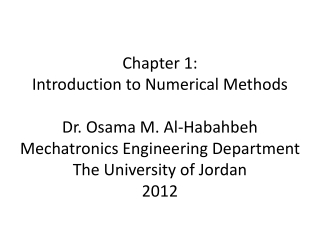DownloadDownload Presentation2- Systems of Linear Algebraic Equations: find values that satisfy the equations.

# 2- Systems of Linear Algebraic Equations: find values that satisfy the equations.

Télécharger la présentation## 2- Systems of Linear Algebraic Equations: find values that satisfy the equations.

- - - - - - - - - - - - - - - - - - - - - - - - - - - E N D - - - - - - - - - - - - - - - - - - - - - - - - - - -
##### Presentation Transcript

1. Chapter 1: Introduction to Numerical MethodsDr. Osama M. Al-HabahbehMechatronics Engineering DepartmentThe University of Jordan2012

2. Numerical Methods are used to formulate mathematical problems as to solve them with arithmetic operations. • Due to the large number of these operations, they are done using computer programs. • Math Review: 1- Roots of Equations: value of parameters to satisfy a single nonlinear equation.

3. 2- Systems of Linear Algebraic Equations: find values that satisfy the equations. 3- Curve Fitting: Fit curve to data points

4. 4- Interpolation: 5- Numerical Integration: I=∫ f(x).dx Area under the curve

5. 6- Ordinary Differential Equations(ODE’s): Initial value problems Boundary value problems eigenvalues 7- Partial Differential equations(PDE’s): Finite difference method Finite Element method

6. Significant Figures - They are figures that can be used with confidence. 0.00001845 0.0001845 4 sig. figs. (SF) 0.001845 3 SF 4 SF 5 SF Round – off error results from elimination of SF`s

7. Accuracy and Precision:

8. Percent relative error(ɛ): ɛ • Error propagation due to iterations.(Build up error) Approximations (Series) Limited sig figs.

9. Solving Non-Linear Equations: - The root of an equation is the value of ‘x’ that makes f(x)=0. - There are two different types of equations: Algebraic, and Transcendental. - A Simple of algebraic functions are the Polynomials. - Example of transcendental functions include Trigonometric, Exponential, and Logarithmic functions.

10. Algebraic Polynomial Transcendental